Question

A tank contains 150 liters of fluid in which 40 grams of salt is dissolved. Brine containing 1 gram of salt per liter is then pumped into the tank at a rate of 3 L/min; the well-mixed solution is pumped out at the same rate. Find the number A(t) of grams of salt in the tank at time t.

1.minhkhoi

The number A(t) of grams of salt in the tank at time t is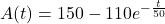Explanation:

Knowing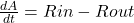First we have to find the Rin and Rout

Rin = (concentration of the salt inflow) * (input rate of brine)

Rin = 1 g/L * 3 L/min = 3 g/L

Rout = (concentration of the salt outflow) * (output rate of brine)

Rout = (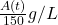) * (3 L/min) =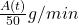Substituting this results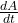= 3 –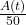–>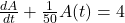Thus, integration factors is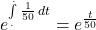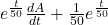A(t) = 4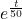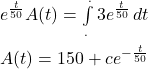Applying the initial conditions

A(0) = 40

c = 150 – 40 = 110

Now, substitute this result in the solution to get